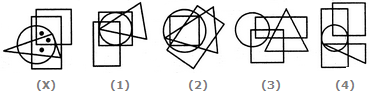# Non Verbal Reasoning - Dot Situation - Discussion

### Discussion :: Dot Situation - Section 2 (Q.No.2)

From amongst the figures marked (1), (2), (3) and (4), select the figure which satisfies the same conditions of placement of the dots as in figure (X).

2.

Select the figure which satisfies the same conditions of placement of the dots as in Figure-X.[A]. 1 [B]. 2 [C]. 3 [D]. 4

Explanation:

In fig. (X), one of the dots lies in the region common to the circle, the square and the rectangle only, another dot lies in the region common to all the four elements - the circle, the square, the triangle and the rectangle and the third dot lies in the region common to the circle, the triangle and the rectangle only. In fig. (1), there is no region common to the circle, the triangle and the rectangle only. In each of the figures (3) and (4) there is no region common to all the four elements - the circle, the square, the triangle and the rectangle. Only fig. (2) consists of all the three types of regions.